# Coulomb's law facts for kids

Kids Encyclopedia Facts

Coulomb's law is a function developed in the 1780s by physicist Charles Augustin de Coulomb. It explains how strong the force will be between two electrostatic charges. Electrostatic means electric charges without any motion.

## Direction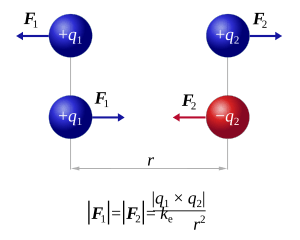This picture shows how Coulomb's Force act; similar charges pushing against each other and opposite charges attract each other

Let's think of two electric charges existing in an empty space. If the two charges are opposite, (+) and (-) charges for example, they will attract each other. And if two charges are both the same, both (+) or both (-) for example, they will push each other. This is similar to how magnets act, as N and S attract each other, and as N and N, S and S push each other.

This is because electric charges make an electric field. If two fields exist in the same space at the same time, then the two fields exert (~ put) force on each other. The force they make on each other is called Coulomb's force or electrostatic force. Coulomb's law explains how big the force will be.

## Scale

Coulomb's law explains the scale between two electric charges. The scale of electrostatic force follows the function below.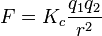$F = {K_c}\frac{q_1q_2}{r^2}$

Coulomb's law explains that the force scale F is relative to ratio of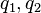$q_1,q_2$,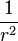$\frac{1}{r^2}$.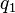$q_1$ and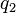$q_2$ are the scales of each electric charge.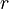$r$ is the distance between the two electric charges. And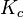$K_c$ has a certain value. It does not change relative to$q_1$,$q_2$ or$r$. While${K_c}$ remains constant, when multiples of$q_1$ and$q_2$ become bigger, the electrostatic force will also get bigger. When the distance$r$ become bigger, the electrostatic force will become smaller to ratio of$\frac{1}{r^2}$.
The exact size of$K_c$ is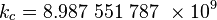\begin{align} k_c &= 8.987\ 551\ 787\ \times 10^9 \\ \end{align}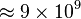$\approx 9 \times 10^9$N m2 C−2 (or m F−1). This constant is called Coulomb's Force Constant or Electrostatic Force Constant.

## Inverse-square law

The relation between the force of pushing or pulling (F) and the distance between the particles ($r$) follows the Inverse-square Law. Inverse-square law means that when the distance$r$ grows bigger, the force gets weaker by the ratio$\frac{1}{r^2}$. Gravitation, Electromagnetic radiation, Sound Intensity also follows this law.

## Images for kidsCoulomb's law Facts for Kids. Kiddle Encyclopedia.